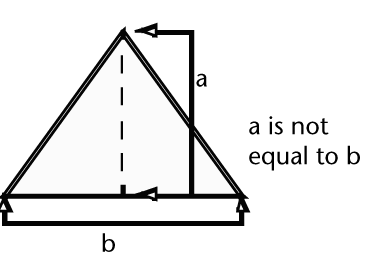Stack the barrels in a triangular arrangement by duplicating the existing row and offsetting the row in both X and Y.Using basic trigonometry, you can calculate the unknown height offset. MEL has a library of built-in functions that include trigonometry functions, to handle these calculations.

NoteTrigonometry functions are ratios that relate two sides of a right angled triangle dependant on one of the angles of the triangle. Trigonometry functions are often used for animation, as trigonometric functions are periodic. Periodic functions cycle their output values after a certain period. These functions can easily create cyclical or repeating animation.